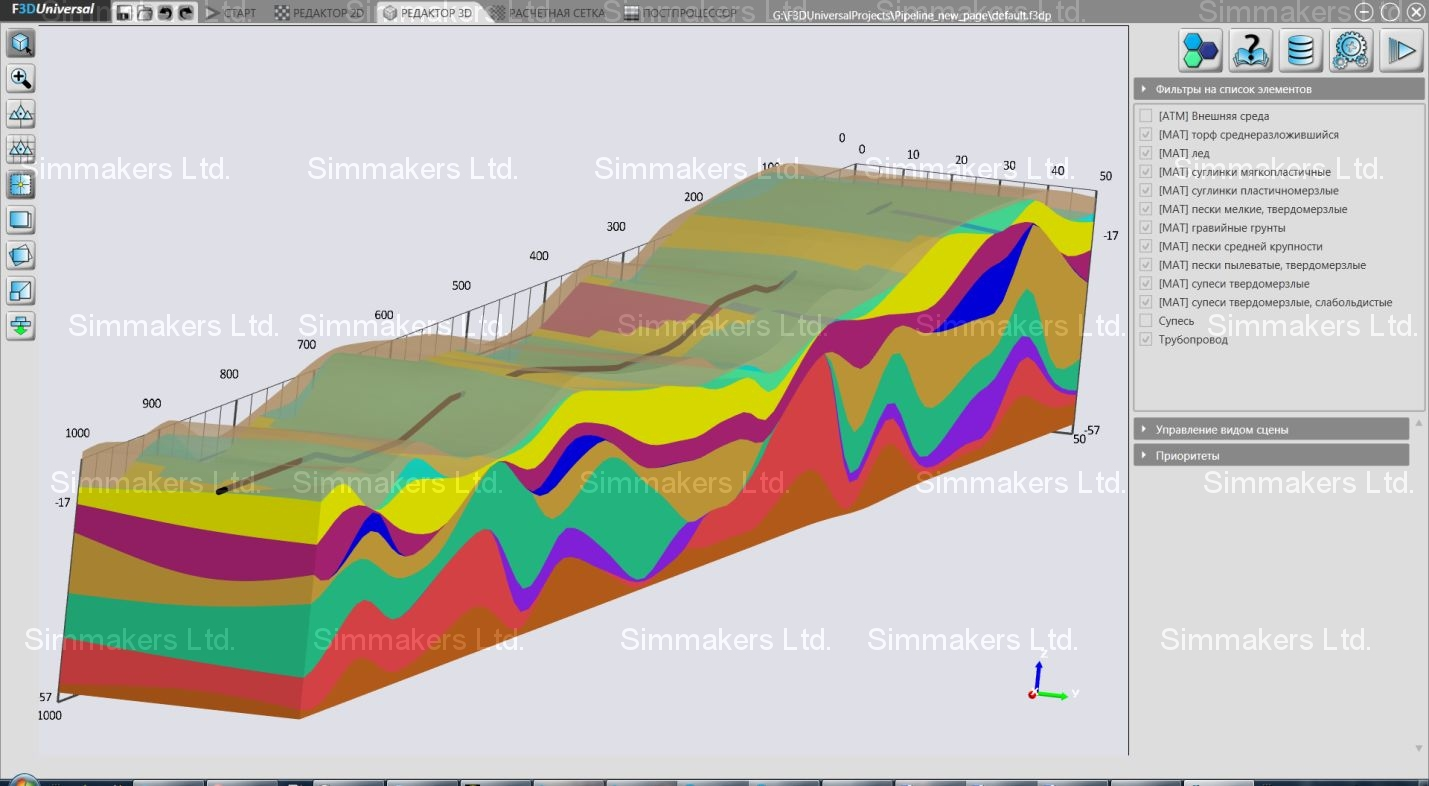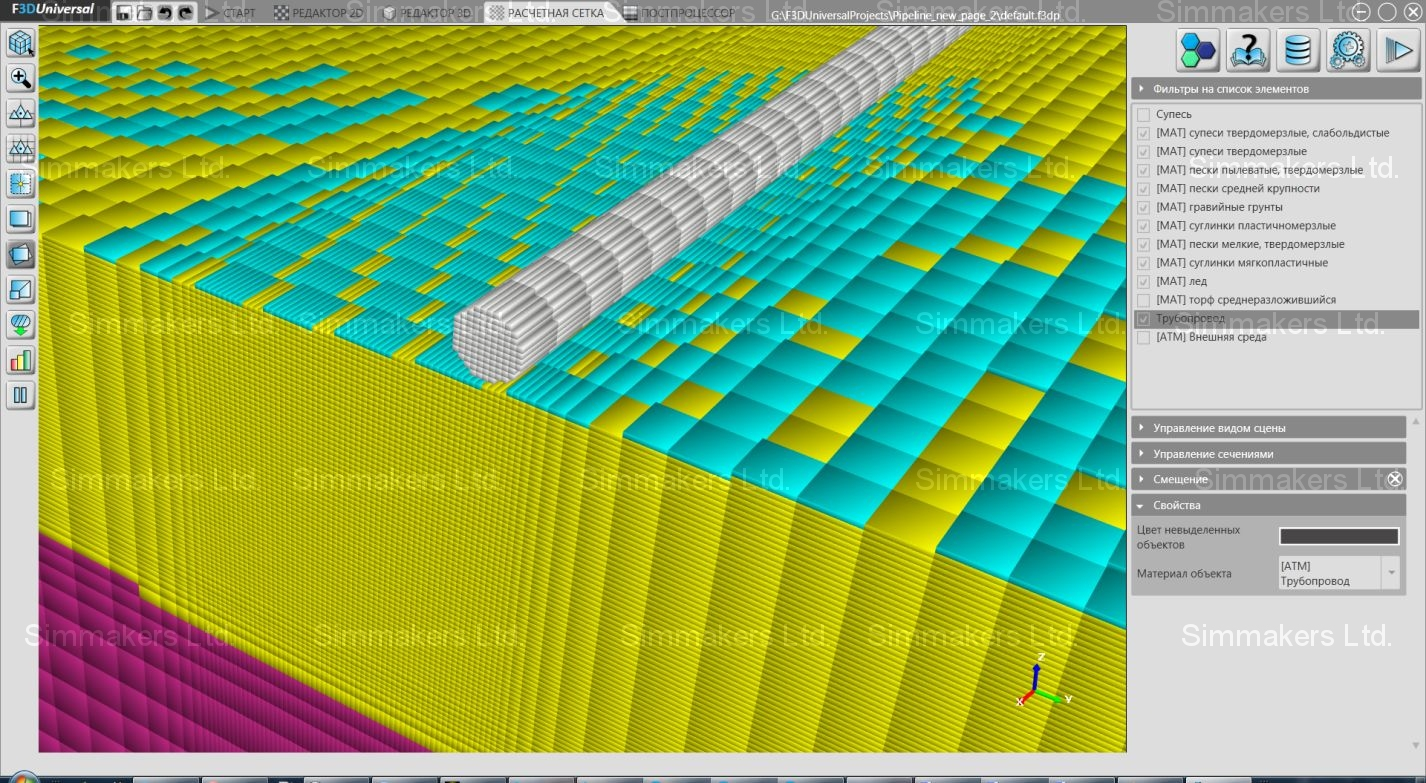When using numerical simulation software, the question “Which mesh is the most suitable for object discretization?” often arises. The same geometry can actually be discretized with a coarse or fine grid mesh, which of course affects the accuracy and speed of numerical computation.

Experts in the field of numerical simulation have analyzed computational meshes created in Frost 3D Universal; a 1-km model of an oil pipeline on permafrost serving as the example. It should be noted that the pipeline geometry ‘bends’ through both the Z and Y planes and some pipeline sections penetrate ice layers. Dimensions of created 3D model: length – 1000 m, width – 50 m, depth – 55 m, diameter – 1.2 m. A thin ice layer under the pipeline at the end of a rectangular area is the main feature of our example. The computational domain was discretized with varying quantity of cells in different versions of Frost 3D Universal , with the results shown below.Pipeline model in 3D

### Computational mesh: 1.8 million cells

• Thin ice layer (in blue) under the pipeline was almost lost during computational mesh creation.
• The 4-m cell size is inappropriate for Stefan problem solving.
• The boundary between thawed and frozen ground was computed with a high degree of inaccuracy; for correct simulation, this model should be discretized with a fine mesh.

### Computational mesh: 4.95 million cellsProgram version: single core CPU. Zoom of ice layers

• Insignificant mesh refinement (if compared with previous one).
• Low quality is noticeable in the thin ice layer mesh, most of which was lost.

### Computational mesh: 19.2 million cellsProgram version: multi-core CPU. Zoom of ice layers

• A 4x increase in the number of cells reduces the maximum cell size up to 1 meter and increases computational mesh accuracy.
• Such increase reduces the inaccuracy of thin ice layer discretization.
• More accurate thermal field computation (near pipe bends in Y axes) is possible with significant mesh refinement.

### Computational mesh: 54.7 million cellsProgram version: multi-core GPU. Zoom of ice layers